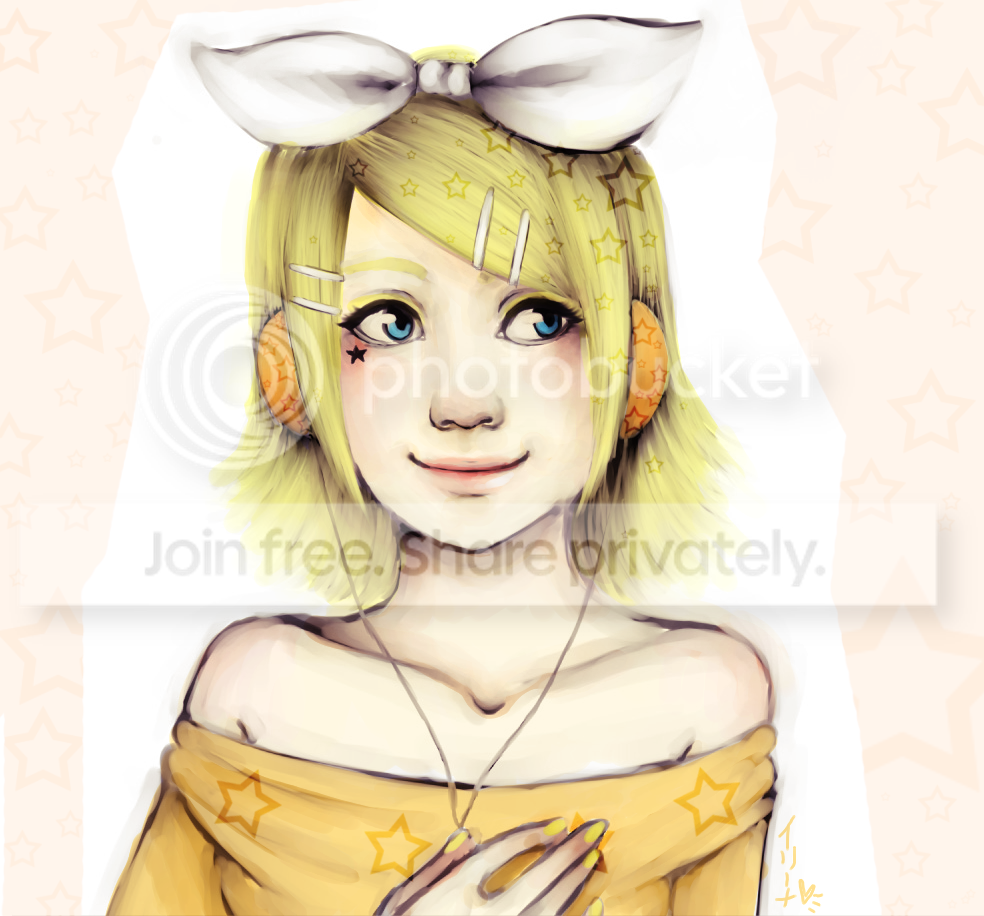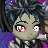# Welcome to Gaia! :: Arenas

••• Artist: Momiji Manju
• Description: Kagamine Rin from vocaloid!

Drawn off the top if my head
Go to my stuff and check out the rest of this series ^^
• Date: 06/26/2010
• Tags: kagamine vocaloid fanart
•••••••• kaiaxxx - 06/26/2010
• wow your awesome wanna be freinds smile )))))))))))))))))))))))))))))))))))))))))))))))))))))))))))))))))))))))))))))))))))))))))) smile ))))))))LOL